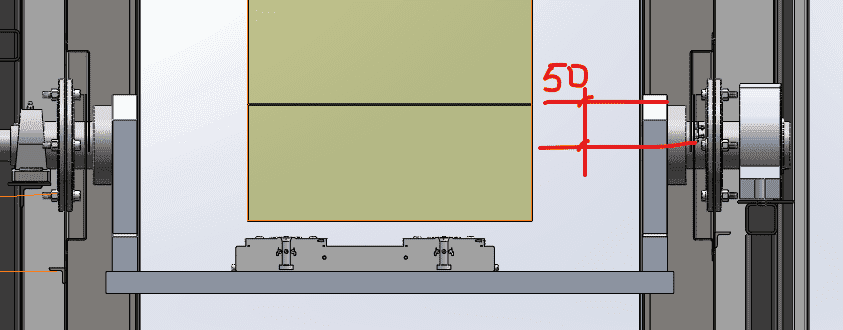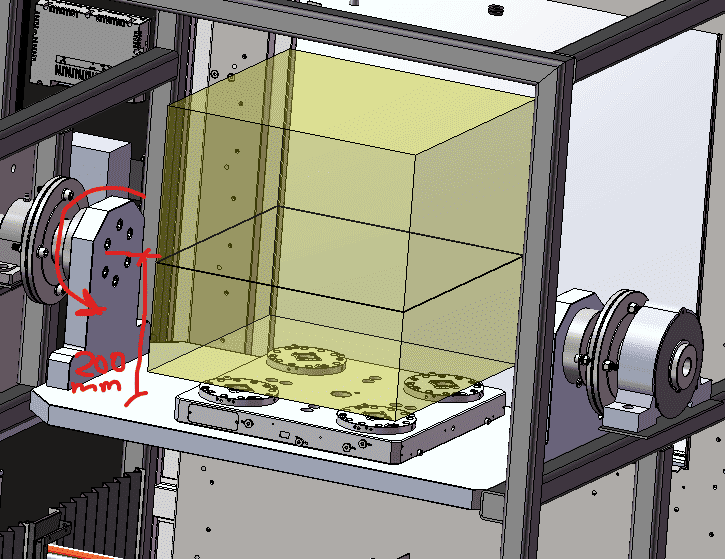Yevhenii
TL;DR Summary
Torque calculation
I'm designing loading mechanism of new machine and need to know what the torque will be.
Here is the sketch of my mechanism.
Distance from centre of shaft to the top of loading table is 200mm. Distance from the centre of mass of workpiece to centre of the shaft 50 mm. Max load is 400 kg. Weight of loading table is 50 kg.
Do I need (at first) calculate moment of inertia and then angular speed, and after I will know what torque i need? Or I just need to multiply force on distance?
This mechanism should rotate to 180 deg. n=14 rpm
ThanksMentor
I'm not clear as to exactly what you are doing. Please show an isometric view in the starting position, and another view partially rotated.

A general procedure for machines that move:
1) Calculate static forces and torques.
2) Calculate accelerations and speeds.
3) Calculate acceleration forces and torques.

If the static forces are sufficiently larger than the dynamic forces, the dynamic forces can be ignored. If the dynamic forces are sufficiently larger than the static forces, the static forces can be ignored. You have to calculate both static and dynamic forces and torques in order find if you need to calculate both.

•Lnewqban
Mentor
Also, are these numbers correct? I must be misunderstanding something...

Yevhenii
Also, are these numbers correct? I must be misunderstanding something...
Weight of loading plate is 50 kg (aluminium). Max weight (of workpiece) is 400 kg.

Yevhenii
I'm not clear as to exactly what you are doing. Please show an isometric view in the starting position, and another view partially rotated.

A general procedure for machines that move:
1) Calculate static forces and torques.
2) Calculate accelerations and speeds.
3) Calculate acceleration forces and torques.

If the static forces are sufficiently larger than the dynamic forces, the dynamic forces can be ignored. If the dynamic forces are sufficiently larger than the static forces, the static forces can be ignored. You have to calculate both static and dynamic forces and torques in order find if you need to calculate both.
I need to know how to calculate torque, if center of mass of workpiece will be higher than shaft center of mass (+50mm).
And if center of mass of workpiece will be on the same axis with shaft, then how do I need to calculate? F*r?

YevheniiHomework Helper
Gold Member
The maximum moment will happen when the table and the work piece have rotated 90°.

The weight of the table times the distance of its center of mass to the axis of rotation (it may be more than 200 mm), creates a moment that opposes the moment created by the weight of the workpiece times the distace from its center of mass to the axis of rotation (it may be different than 50 mm),

Installing enough counter-balance on the botton of the table could eliminate the resulting moment; although it would change the moment of inertia some.

Last edited:
Mentor
Now that you have calculated the static moment, the next step is to calculate the torque to accelerate and decelerate. A typical step by step process is as follows:

Step 1: Decide how you are going to drive the unit. Some (not all) possibilities include induction motor and gearbox with contactor, induction motor and gearbox with VFD drive, servomotor and gearbox, hydraulic rotary actuator with solenoid valve, hydraulic rotary actuator with servovalve and servo controller. Each of those has advantages and disadvantages. Some of those have full ability to control acceleration, others do not. Your OP indicates that you have very little experience with this, so expect to spend at least 100 hours of concentrated effort on this step. How accurately do you need to rotate it - +/- 1 degree, +/- 0.1 degree, +/- 0.01 degree?

Step 2: Calculate the acceleration, velocity, and position vs time curves for your chosen drive.

Step 3: Check the peak velocity against gear ratio, RPM, or flow rate (if hydraulic). Adjust as necessary.

Step 4: Calculate acceleration torque from the mass moment of inertia.

Step 5: Plot acceleration torque and static torque on same axes. The sum of those two torques is the total drive torque.

Step 6: Check peak torque against the drive specifications. Adjust if necessary.

And yes, this is an iterative process. Machine design is like that.

•Lnewqban
Yevhenii
Now that you have calculated the static moment, the next step is to calculate the torque to accelerate and decelerate. A typical step by step process is as follows:

Step 1: Decide how you are going to drive the unit. Some (not all) possibilities include induction motor and gearbox with contactor, induction motor and gearbox with VFD drive, servomotor and gearbox, hydraulic rotary actuator with solenoid valve, hydraulic rotary actuator with servovalve and servo controller. Each of those has advantages and disadvantages. Some of those have full ability to control acceleration, others do not. Your OP indicates that you have very little experience with this, so expect to spend at least 100 hours of concentrated effort on this step. How accurately do you need to rotate it - +/- 1 degree, +/- 0.1 degree, +/- 0.01 degree?

Step 2: Calculate the acceleration, velocity, and position vs time curves for your chosen drive.

Step 3: Check the peak velocity against gear ratio, RPM, or flow rate (if hydraulic). Adjust as necessary.

Step 4: Calculate acceleration torque from the mass moment of inertia.

Step 5: Plot acceleration torque and static torque on same axes. The sum of those two torques is the total drive torque.

Step 6: Check peak torque against the drive specifications. Adjust if necessary.

And yes, this is an iterative process. Machine design is like that.
Thanks for help. I calculated static moment and its much more larger than torque I calculated through moment of Inertia.
As I understood, bigger value will be the peak moment?
I will use worm gearbox, ratio 100. Output torque is 750 Nm

Last edited: